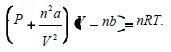# CBSE Class 11 Chemistry States Of Matter Notes Set E

Download CBSE Class 11 Chemistry States Of Matter Notes Set E in PDF format. All Revision notes for Class 11 Chemistry have been designed as per the latest syllabus and updated chapters given in your textbook for Chemistry in Standard 11. Our teachers have designed these concept notes for the benefit of Grade 11 students. You should use these chapter wise notes for revision on daily basis. These study notes can also be used for learning each chapter and its important and difficult topics or revision just before your exams to help you get better scores in upcoming examinations, You can also use Printable notes for Class 11 Chemistry for faster revision of difficult topics and get higher rank. After reading these notes also refer to MCQ questions for Class 11 Chemistry given our website

## Revision Notes for Class 11 Chemistry States Of Matter

Class 11 Chemistry students should refer to the following concepts and notes for States Of Matter in standard 11. These exam notes for Grade 11 Chemistry will be very useful for upcoming class tests and examinations and help you to score good marks

### States Of Matter Notes Class 11 Chemistry

Important points

1. Change in state : It is over all effect of Intermolecular forces, molecular Interactional energy & thermal energy:

2. Measurable properties of gases : P,V, T, n, Viscosity, specific heat are some measurable properties.

3. Gas Laws : The quantitative relationship b/w any two of the variables (V, n, P,T) when other two are constant.

4. Boyle’s Law : The pressure of fixed msss of gas varies inversely with the volume at constant T. P α 1/V(n,T const.) P1V1 = P2 V2

5. Charle’s Law : At constant P, the volume of fixed amount of gas varies directly with its absolute temperature.

V α T or V/T=cons tan t,V1/T1=V2/T2

6. Gay lussac’s Law : At constant V, The pressure of fixed amount of gas varies directly with its absolute temperature.

P α T or P/T const,P1/T1=P2/T2

7. Ideal gas equation : The relationship b/w P, V and T by Gas Laws PV= nRT.

8. Avogadro’s Law : At given T and P, the volume of gas varies directly to the amount of gas . V α n ( P, T constant)

9. Dalton’s Law of partial persure : The pressure enerted by a mixture of non reacting gases is equal to the sum of their partial pressure at constant (V,T) P (total ) = P1 + P2 + P3 + ………. (T, V, constant)

10. Kinetic Molecular theory :

a. Gases consist of large number of identical particles (atoms or molecules) that are so small that the actual volume of the molecules is negligible in comparison to the empty space between them.

b. There is no force of attraction between the particles of a gas at ordinary temperature and pressure

c. Particles of a gas are always in constant and random motion

d. Pressure is exerted by the gas as a result of collision of the particles with the walls of the container

e. Collisions of gas molecules are perfectly elastic

f. At any particular time, different particles in the gas have different speeds and hence different kinetic energies

g. Average kinetic energy of the gas molecules is directly proportional to the absolute temperature

11. Real Gases : The gases which deviates from Ideal behavior at higher pressure and low temperature b/c of force of attraction b/w molecules increases .

12. Compressibility factor (Z) : It determine extent of devation of real gases from Ideal gas behavior : Z = gas Z=1, for Nonideal gas Z< 1, Z > 1

13. Vander waal’s Equation :14. Critical Temperature : (Tc) The temperature above which a gas cannot be liquefied whatever high pressure may be

15. Critical Pressure : The minimum pressure required to liquity a gas at its critical temperatur

16. Critical b: The volume of 1 mole of gat at Tc,

17. Super cooled liquids : The liquids which are cooled to a temperature below its freezing point without freezing .

18. Elastic Collision : The collisions in which no loss of K.E. only there is transfer of energ

19. Vapour pressure : The equilibrium pressure by vapour of liquid in a container at given temperature (T)

20. At higher altitude : The b. of water decreases b/c the atmospheric pressure is less than one atmosphere.

21. Surface Tension (V) : It is force acting per unit length perpendicular to the line drawn on the surface : (Nm-1) : It decreases with increases in T, it increases with increase in external pressure, b/c of it falling drops of liquid are spherical, liquid in capillary tube rises

22. Viscosity (η) : It is resistance offered to the flow of liquid due to friction b/w layer of fluids . F = n.A.* dv/dn

23. Effect of T & P on viscosity : It decreases with increase in T, and increases with increase in P.

24Low M.P. & B.P. of molecular liquids is due to low magnitude of molecular interaction energy.